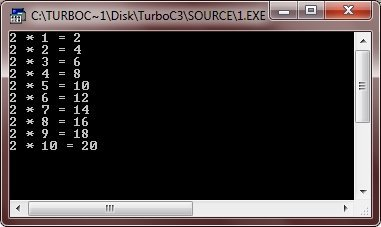# C break Statement

In C programming, the break statement has the following two uses:

• use break statement to terminate a case in the switch statement
• use break statement to force immediate termination of a loop, bypassing the normal loop conditional test

Now let's go through the following example program that illustrates the concept of break statement in C.

## C break Statement Example

Here is an example program of that uses the break statement in C programming.

```/* C break Statement Example
* This program illustrates
* the concept of break statement in C
*/

#include<stdio.h>
#include<conio.h>
void main()
{
int i;
int num = 2;
clrscr();

for(i=1; i<50; i++)
{
if(i==11)
break;
printf("%d * %d = %d\n", num, i, num*i);
}

getch();
}```

This program prints the table of 2. Here is the sample run of this program.You can also use break statement in the switch statement. To see how, refer calculator program.

Tools
Calculator